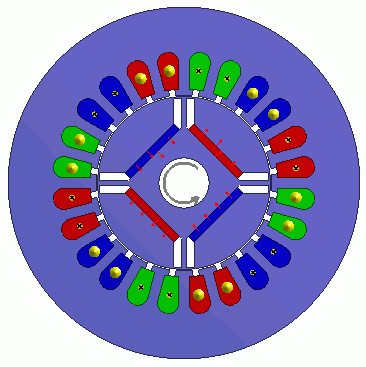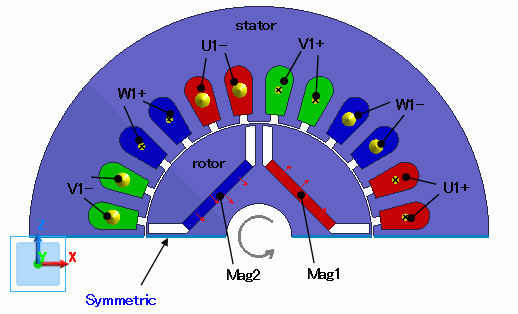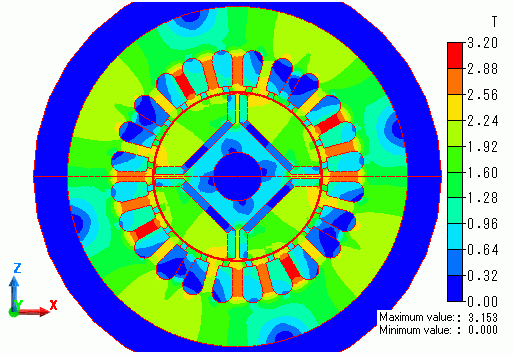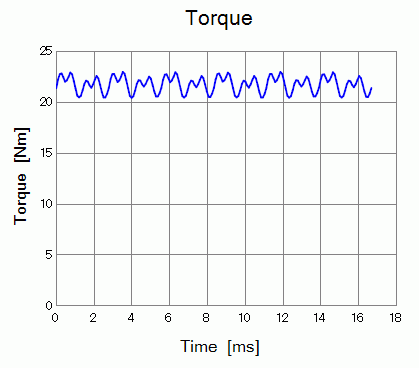﻿ Torque Analysis of IPM Motor (Current Source)Examples | Product | Murata Software Co., Ltd.Example7Torque Analysis of IPM Motor (Current Source)General

• Torque characteristics of IPM motor (Interior Permanent Magnet Motor) is analyzed when the electricity is applied.
The model is a permanent magnet synchronous motor with magnets built in a rotor.

• The form of model is same as Exercise 1, but the three-phase AC source is used in the external circuit.
(Exercise 1 uses the three-phase AC voltage source.)

• The analysis is coupled with the external circuit.

• The homogenizing method is applied to simulate the layer structure of the electromagnetic plates of steel for the core.

• The torque and the magnetic flux density are solved.

• Unless specified in the list below, the default conditions will be applied.

• In this exercise, the model is half period symmetric for your easy understanding.
Fast calculation is possible by making this model 1/4 period symmetric.
Obtain the project file of 1/4 period symmetric model. (Save the project file before open)

Analysis Conditions

 Item Setting Solver Magnetic Field Analysis [Luvens] Analysis Space 2D Analysis Type Transient Analysis Unit mm Options Select External Circuit Coupling. Select Rotating machinery.   [Conversion] Model Thickness: 30×10^-3[m] Select Partial Model. Number of Divisions of the Whole Model: 2 Circuit Configuration Number of Series: 1 Circuit Configuration Number of parallels: 2 Select Convert the result to the whole model and output it.

The Rotating Machinery tab is set as follows.

 Tab Setting Item Setting Rotating Machinery Rotational Movement Select Constant Velocity Number of Rotations: 1800[r/min] Rotor’s Initial Rotation Position: 0[deg] Number of Slide Mesh Divisions Circumferential Division Angle: 1.0[deg] Rotation per Step: 1[mesh] Number of Slide Mesh Layers: 3

External circuit is as follows.

60[Hz] three-phase AC is applied.

Set the Mesh Tab as follows.

 Tab Setting Item Setting Mesh Meshing Setup Automatically set the general mesh size: Deselect General mesh size: 1[mm] Ambient Air Creation Select Create ambient air automatically Ambient Air Scale: 1.2

The Transient Analysis tab is set up as follows.

The umber of steps is 180, circumferential division angle is 1.0[deg], and rotation per step is 1[mesh]. The rotation up to 180 degrees (=180*1.0*1) is analyzed.

Tab

Setting Item

Setting

Transient Analysis

Time step

Automatic

Table

 Number Calculation steps Output steps 1 180 1

Graphical Objects

A rotor core and magnet are placed in the center. A stator and coils are placed around them.

The motor has 4 poles.

This is a 2D model analysis. By utilizing the symmetry of the analysis model, it is made to be a half period symmetric model.

Rotation period boundary (symmetric) is set.

“Ambient Air Creation” is selected.

• In this exercise, the model is half period symmetric for your easy understanding. Fast calculation is possible by making this model 1/4 period symmetric.
In the quarter model, the number of series in the circuit is 2 and the resistance value of FEM coil in the external circuit is half of the half model.Body Attributes and Materials

 Body Number/Type Body Attribute Name Material Name 14/Sheet Mag1 mag 15/Sheet Mag2 mag 13/Sheet Rotor core 37/Sheet Stator core 39/Sheet U1+ Cu 40/Sheet U1+ Cu 41/Sheet W1- Cu 42/Sheet W1- Cu 43/Sheet V1+ Cu 44/Sheet V1+ Cu 45/Sheet U1- Cu 46/Sheet U1- Cu 47/Sheet W1+ Cu 48/Sheet W1+ Cu 49/Sheet V1- Cu 50/Sheet V1- Cu

The body attribute is set up as follows.

For the core, the homogenizing method is selected to simulate the layered steel plates.

 Body Attribute Name Tab Setting Mag1 Direction Vector: X=1, Y=0, Z=1 Stator/Rotor/Air Rotor Mag2 Direction Vector: X=1, Y=0, Z=-1 Stator/Rotor/Air Rotor Rotor Layer Select Take layer into account Space: 97[%] Layer direction vector: X=0, Y=1, Z=0 Stator/Rotor/Air Rotor Stator Layer Select Take layer into account Space: 97[%] Layer direction vector: X=0, Y=1, Z=0 Stator/Rotor/Air Stator U1- Current Waveform: External circuit coupling Coil name on the circuit: U1 Turns: 35[Turns] Direction: – Y direction Stator/Rotor/Air Stator U1+ Current Waveform: External circuit coupling Coil name on the circuit: U1 Turns: 35[Turns] Direction: +Y direction Stator/Rotor/Air Stator V1- Current Waveform: External circuit coupling Coil name on the circuit: V1 Turns: 35[Turns] Direction: – Y direction Stator/Rotor/Air Stator V1+ Current Waveform: External circuit coupling Coil name on the circuit: V1 Turns: 35[Turns] Direction: +Y direction Stator/Rotor/Air Stator W1- Current Waveform: External Circuit Coupling Coil name on the circuit: W1 Turns: 35[Turns] Direction: – Y direction Stator/Rotor/Air Stator W1+ Current Waveform: External circuit coupling Coil name on the circuit: W1 Turns: 35[Turns] Direction: +Y direction Stator/Rotor/Air Stator

The material properties are set as follows.

Material Name

Tab

Properties

Cu

Conductivity

Conductivity Type: Conductor

Conductivity: 5.977×10^7[S/m]

mag

Permeability

Material Type: Permanent magnet

Magnet

Magnetization Characteristic Type: Linear

Magnetization Strength: 1.25

Relative Permeability: 1.05

core

Permeability

Magnetization Characteristic Type: Select B-H curve

B-H Curve Table

 Magnetic Field [A/m] Magnetic Flux Density [T] 0 0 58 0.42 90 0.8 180 1.19 380 1.37 1100 1.48 2000 1.55 3000 1.608 11000 1.81 20000 1.91

Boundary Condition

Half period symmetric boundary is set.

 Boundary Condition Name/Topology Tab Boundary Condition Type Setting Symmetric Symmetry/Continuity Periodic Rotation Period (1 period)

Results

The distribution of the magnetic flux density at the rotation angle of 0[deg] is shown below.The diagram below shows time-torque characteristics.

About 20[N*m] of torque is obtained.﻿